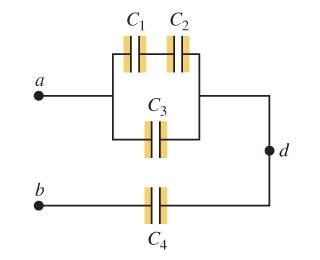# Energy stored in Capacitor Network

## Homework Statement

A potential difference Vab = 46.0 V is applied across the capacitor network of the following figure.If C1=C2=4.00μF and C4=8.00μF, what must the capacitance C3 be if the network is to store 2.80×10−3 J of electrical energy?

## Homework Equations

U=(1/2)CV^2
(1/C) = (1/c1) + (1/c2) + ... capacitors in series
C = C1 + C2 + ... capacitors in parallel

## The Attempt at a Solution

U = (1/2)CV^2
2.80×10−3 = (1/2)C(46^2)
C = 2.6 μF

Capacitance of C1 and C2 = 1/(1/4 + 1/4) = 2 μF
Total capacitance:
1/2.6 = 1/(2+C3) + 1/8
C3 = 1.85 μF

I can't figure out why this answer is incorrect. Help much appreciated!

•